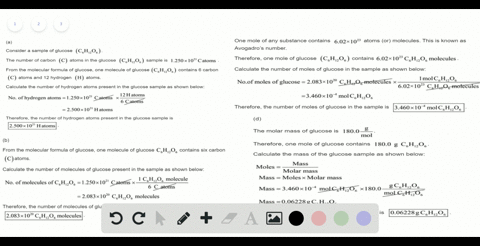Sign up for our free STEM online summer camps starting June 1st!View Summer Courses## Discussion

You must be signed in to discuss.

## Video Transcript

Okay, so in this problem, first we have to find out the Moler mass off aspartame, which has a molecule or formula off this. So the manners for the Moler man's off. This compound is simply the sum of the Mormons off each atom present here. And we can do that by just simply adding first year. I don't know formula here. See Fortin, age eighteen into Oh, fine. That means the solar mass off this compound will be weapons zero one, which is the monuments of carbon. And we have fourteen Carbone. They're so will multiply this BA fourteen, then elements of fighters and is one point or eat. We have eighteen had risen, multiplied by eighteen. Then we have nitrous and nitrous and has a molar mass off fourteen point zero zero six seven. And we have two night Rosen. And finally we have oxygen, which has a more mouths off sixteen, and we have five oxygen. So just doing that the total will give us a fellow off two ninety four point tree, which is in Graham Carmel. So this is them molar mass of s Pardon our this compound. Now, in the next problem, we have to find out how many more self A spark tamer presenting one milligram a spartan. So we have already calculated the molar mass. That means Ah, one more of his program contents. Thiss Ingraham of a Spartan. You can write it here. We converted the other way. Make two ninety four point three. Graham is contained in one more. That means one milligram or one times ten to the four minus three. Graham is contending one time student of four minus three, Graham over two ninety four point dream cramp our mullahs. And this will give us a bell off three point four zero times ten to the minus six more? No. So this is them. Number of malls off Esper team, which is present in one milligram of expertise. Now, the next part, we have to find out how many molecules of us partying are presenting one milligram of a spartan. So we have find out Ah, the number ofthe malls, office per team for one milligram. Now we know that one more off any substance or a monocle or substance Contents six point zero times ten to the bar. Twenty three molecules. That means three point four times end of the bar minus six, which is the number of malls. For this mess, this must mall will contain three point four times ten door minus six time, six point zero toe times ten to the work twenty three molecules and you'LL give us a value off two point Oh, four time stunt with the bar eighteen molecules. So this is the number of molecules which is contained in this much number off balls of aspartame. And again, since this is the number of most of us parting for one milligrams per team, that means this much more nickels are contending one time. So there were minus three graham or one milligram off a spartan one milligram aspartame contains this number off Monica ls and finally had to find out how many hydrogen atoms are presenting the same mass of aspartame. So we have calculated how many molecules we have in one milligram off aspartame. Now we have to find out how many high treason atom we have in one milligram of fast part in so as they know that from the formula, we can see that one molecule off aspartame contains eighteen atoms off hydrogen. That means this much molecules off aspartame. When will contain simply this time eighteen thousand atom, These times eighteen atoms of hydrogen. And if we just multiply this by eighteen, we will get a fellow off three point six eight times into the barn nineteen. So this is the number off hydrogen atoms two point six eight times ten to the one nineteen. Hydrogen atoms are present in one milligram off S. Martin.